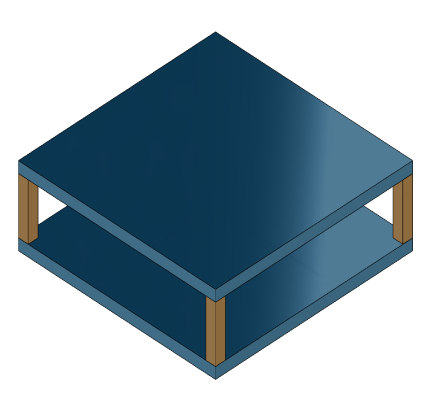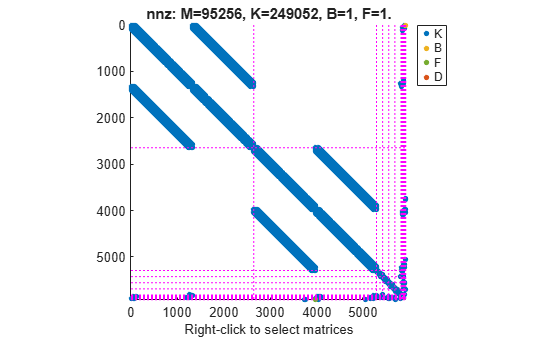Rigid Assembly of Model Components

This example shows how to specify rigid physical coupling between the components of a structural model. Consider a structure that consists of two square plates connected with pillars at each vertex as depicted in the figure below. The lower plate is connected rigidly to the ground while the pillars are connected rigidly to each vertex of the square plates.platePillarModel.mat contains the sparse matrices for pillar and plate model. Load the finite element model matrices contained in platePillarModel.mat and create the sparse second-order state-space model representing the above system.

sys = ...
mechss(M1,[],K1,B1,F1,'Name','Plate1') + ...
mechss(M2,[],K2,B2,F2,'Name','Plate2') + ...
mechss(Mp,[],Kp,Bp,Fp,'Name','Pillar3') + ...
mechss(Mp,[],Kp,Bp,Fp,'Name','Pillar4') + ...
mechss(Mp,[],Kp,Bp,Fp,'Name','Pillar5') + ...
mechss(Mp,[],Kp,Bp,Fp,'Name','Pillar6')
Sparse continuous-time second-order model with 1 outputs, 1 inputs, and 5820 degrees of freedom.

Use "spy" and "showStateInfo" to inspect model structure.
Type "properties('mechss')" for a list of model properties.
Type "help mechssOptions" for available solver options for this model.

The resultant model sys has 5820 degrees of freedom, one input and one output.

Use showStateInfo to examine the components of the mechss model object.

showStateInfo(sys)
The state groups are:

Type        Name      Size
----------------------------
Component    Plate1     2646
Component    Plate2     2646
Component    Pillar3     132
Component    Pillar4     132
Component    Pillar5     132
Component    Pillar6     132

The named components are listed in the command window with their respective sizes.

Now, load the interfaced DOF index data from dofData.mat and use interface to create the physical connections between the two plates and the four pillars. dofs is a 6x7 cell array where the first two rows contain DOF index data for the first and second plate while the remaining four rows contain index data for the four pillars.

Now, specify rigid couplings between the plates and the pillars.

for i=3:6
sys = interface(sys,"Plate1",dofs{1,i},"Pillar"+i,dofs{i,1});
sys = interface(sys,"Plate2",dofs{2,i},"Pillar"+i,dofs{i,2});
end

Specify rigid connection between the bottom plate and the ground.

sysCon = interface(sys,"Plate2",dofs{2,7})
Sparse continuous-time second-order model with 1 outputs, 1 inputs, and 5922 degrees of freedom.

Use "spy" and "showStateInfo" to inspect model structure.
Type "properties('mechss')" for a list of model properties.
Type "help mechssOptions" for available solver options for this model.

Notice that the model now contains 5922 degrees of freedom. The extra DOFs are a result of the specific rigid interfaces.

interface uses 'dual assembly' formulation to connect the components. In the concept of dual assembly, the global set of degrees of freedom (DoFs) $\mathit{q}$ is retained and the physical coupling is expressed as consistency and equilibrium constraints at the interface. For rigid connections, these constraints are of the form:

$Bq=0,\phantom{\rule{0.2777777777777778em}{0ex}}\phantom{\rule{0.2777777777777778em}{0ex}}\phantom{\rule{0.2777777777777778em}{0ex}}\phantom{\rule{0.2777777777777778em}{0ex}}\phantom{\rule{0.2777777777777778em}{0ex}}\phantom{\rule{0.2777777777777778em}{0ex}}\phantom{\rule{0.2777777777777778em}{0ex}}\phantom{\rule{0.2777777777777778em}{0ex}}\phantom{\rule{0.2777777777777778em}{0ex}}\phantom{\rule{0.2777777777777778em}{0ex}}g=-{B}^{T}\lambda$

where $\mathit{g}$ is the vector of internal forces at the interface, and the matrix $\mathit{B}$ is permutable to $\left[\mathit{I}\text{\hspace{0.17em}}\text{\hspace{0.17em}}-\mathit{I}\right]$. For a pair of matching DOFs with indices ${\mathit{i}}_{\mathit{i}}$, ${\mathit{i}}_{2}$ where ${\mathit{i}}_{\mathit{i}}$ selects a DOF in the first component while ${\mathit{i}}_{2}$ selects the matching DOF in the second component, $Bq=0$ enforces consistency of displacements:

$q\left({i}_{1}\right)=q\left({i}_{2}\right)$

while $g=-{B}^{T}\lambda$ enforces equilibrium of the internal forces g at the interface:

$g\left({i}_{1}\right)+g\left({i}_{2}\right)=0$.

Combining these constrains with the uncoupled equations $M\underset{}{\overset{¨}{q}}+C\underset{}{\overset{˙}{q}}+Kq=f+g$ leads to the following dual assembly model for the coupled system:

$\left[\begin{array}{cc}M& 0\\ 0& 0\end{array}\right]\left[\begin{array}{c}\underset{}{\overset{¨}{q}}\\ \lambda \end{array}\right]+\left[\begin{array}{cc}C& 0\\ 0& 0\end{array}\right]\left[\begin{array}{c}\underset{}{\overset{˙}{q}}\\ \lambda \end{array}\right]+\left[\begin{array}{cc}K& {B}^{T}\\ B& 0\end{array}\right]\left[\begin{array}{c}q\\ \lambda \end{array}\right]=\left[\begin{array}{c}f\\ 0\end{array}\right]$

Use showStateInfo to confirm the physical connections.

showStateInfo(sysCon)
The state groups are:

Type            Name         Size
-----------------------------------
Component        Plate1        2646
Component        Plate2        2646
Component       Pillar3         132
Component       Pillar4         132
Component       Pillar5         132
Component       Pillar6         132
Interface    Plate1-Pillar3      12
Interface    Plate2-Pillar3      12
Interface    Plate1-Pillar4      12
Interface    Plate2-Pillar4      12
Interface    Plate1-Pillar5      12
Interface    Plate2-Pillar5      12
Interface    Plate1-Pillar6      12
Interface    Plate2-Pillar6      12
Interface    Plate2-Ground        6

You can use spy to visualize the sparse matrices in the final model. Choose between the matrices to be displayed using the display menu that can be accessed by right-clicking on the plot.

spy(sysCon)Acknowledgements

The data set for this example was provided by Victor Dolk from ASML.